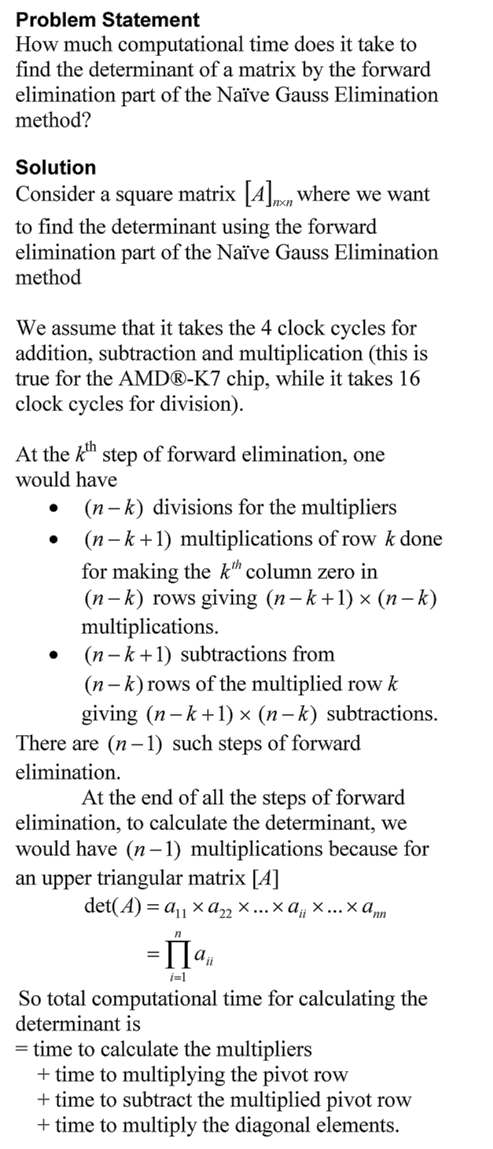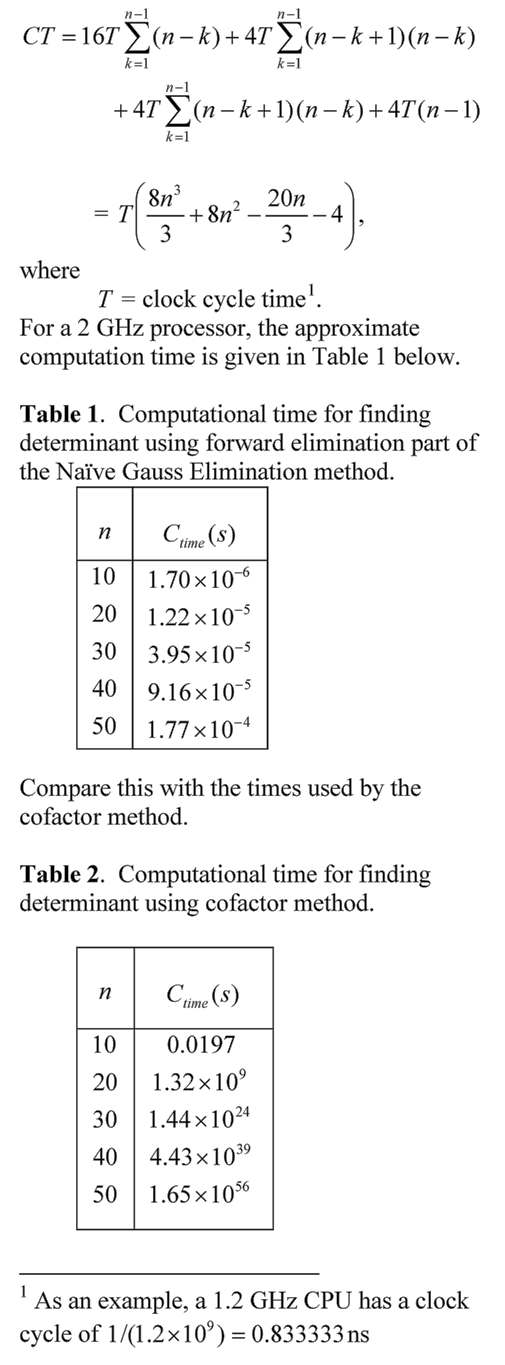## Computational Time to Find Determinant Using Gaussian Elimination

The time it would take to find the determinant of a matrix using the Gaussian Elimination is many-many orders less than when the cofactor method is used.  In this blog, we derive the formula for a typical amount of computational time it would take to find the determinant of a nxn matrix using the forward elimination part of the Naive Gauss Elimination method.  The time is compared with that using the cofactor method.This post is brought to you by Holistic Numerical Methods: Numerical Methods for the STEM undergraduate at http://nm.mathforcollege.com, the textbook on Numerical Methods with Applications available from the lulu storefront, the textbook on Introduction to Programming Concepts Using MATLAB, and the YouTube video lectures available at http://nm.mathforcollege.com/videos.  Subscribe to the blog via a reader or email to stay updated with this blog. Let the information follow you.

## Does the solve command in MATLAB not give you an answer?

Recently, I had assigned a project to my class where they needed to regress n number of x-y data points to a nonlinear regression model y=exp(b*x).  However, they were NOT allowed to transform the data, that is, transform data such that linear regression formulas can be used to find the constant of regression b.  They had to do it the new-fashioned way: Find the sum of the square of the residuals and then minimize the sum with respect to the constant of regression b.

To do this, they conducted the following steps

1. setup the equation by declaring b as a syms variable,
2. calculate the sum  of the square of the residuals using a loop,
3. use the diff command to set up the equation,
4. use the solve command.

However, the solve command gave some odd answer like log(z1)/5 + (2*pi*k*i)/5.  The students knew that the equation has only one real solution – this was deduced from the physics of the problem.

We did not want to set up a separate function mfile to use the numerical solvers such as fsolve.  To circumvent the setting up of a separate function mfile, we approached it as follows.  If dbsr=0 is the equation you want to solve, use

F = vectorize(inline(char(dbsr)))
fsolve(F, -2.0)

What char command does is to convert the function dbsr to a string, inline constructs it to an inline function, vectorize command vectorizes the formula (I do not fully understand this last part myself or whether it is needed).

This post is brought to you by Holistic Numerical Methods: Numerical Methods for the STEM undergraduate at http://nm.mathforcollege.com, the textbook on Numerical Methods with Applications available from the lulu storefront, the textbook on Introduction to Programming Concepts Using MATLAB, and the YouTube video lectures available athttp://nm.mathforcollege.com/videos.  Subscribe to the blog via a reader or email to stay updated with this blog. Let the information follow you.